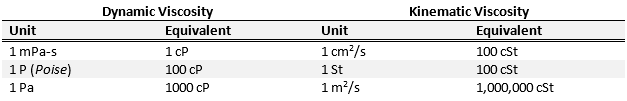Units of Viscosity: Part IThe most commonly used unit for dynamic viscosity is centipoise (cP), which is equivalent to 0.01 Poises (P). This unit is used in honor of French physicist Jean Léonard Marie Poiseuille (1797-1869) who worked with Gotthilf Hagen on the widely known Hagen-Poiseuille’s law which applies to laminar flow through pipes. It is not a coincidence that the viscosity of distilled water at 20°C was used to define 1 cP. In order to give you an idea of the viscosity of some conventional fluids we have collected their viscosities in Table 1. The SI unit for viscosity is the Pascal-second (Pa-s), which corresponds to the force (N) per unit area (m2) divided by the rate of shear (s-1). However, since the viscosity of most fluids is below 1 Pa-s (See table 1), the cP equivalent or milipascal-second (mPa-s) is often used.

Table 1. Viscosity of Common FluidsKinematic viscosity is often measured in centistokes (cSt), which is equivalent to 0.01 stokes (St). You guessed it! This one is named after Irish mathematician Sir George Gabriel Stokes (1819 -1903) who among other contributions to fluid mechanics helped develop Navier-Stokes equation for the conservation of momentum.One St is equivalent to one poise divided by density in g/cm3. SI units for kinematic viscosity are square meters by second (m2/s) but again due to the magnitude of the viscosity of most common fluids square centimeter by second (cm2/s) which is equivalent to 100 cSt is used. In table 2, we present most common units for viscosity conversion.

Table 2. Conversion between common viscosity units.These are the most basic units used for viscosity. Stay tuned for more posts regarding units that are specific to some applications. If you are interested in learning more basics about viscosity read our post on the difference between Newtonian and non-Newtonian Fluids.